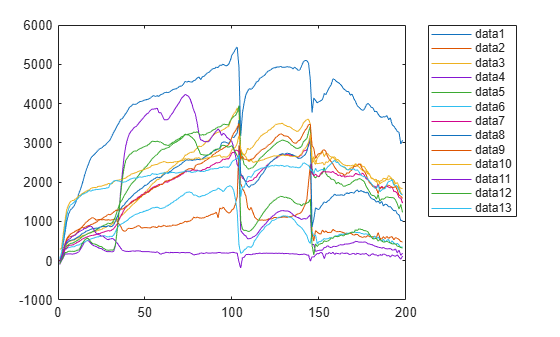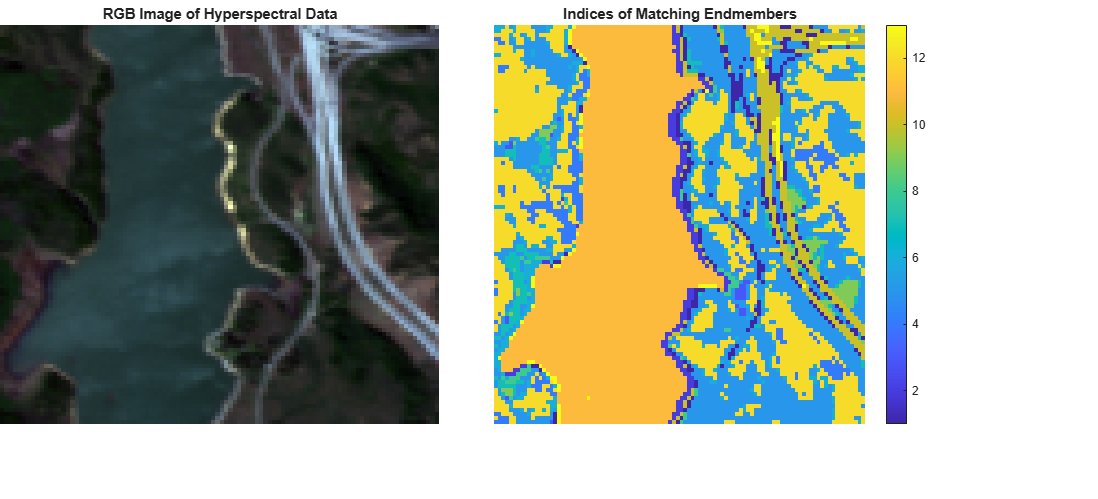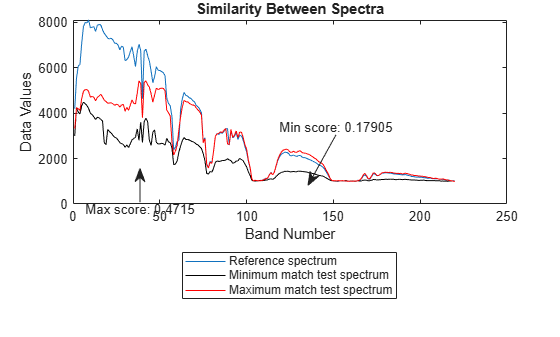# sam

Measure spectral similarity using spectral angle mapper

Since R2020a

## Syntax

``score = sam(inputData,refSpectra)``
``score = sam(testSpectra,refSpectra)``

## Description

example

````score = sam(inputData,refSpectra)` measures the spectral similarity between the spectra of each pixel in the hyperspectral data `inputData` and the specified reference spectra `refSpectra` by using the spectral angle mapper (SAM) classification algorithm. Use this syntax to identify different regions or materials in a hyperspectral data cube.```

example

````score = sam(testSpectra,refSpectra)` measures the spectral similarity between the specified test spectra `testSpectra` and reference spectra `refSpectra` by using the SAM classification algorithm. Use this syntax to compare the spectral signature of an unknown material against the reference spectra or to compute spectral variability between two spectral signatures. NoteThis function requires the Image Processing Toolbox™ Hyperspectral Imaging Library. You can install the Image Processing Toolbox Hyperspectral Imaging Library from Add-On Explorer. For more information about installing add-ons, see Get and Manage Add-Ons.The Image Processing Toolbox Hyperspectral Imaging Library requires desktop MATLAB®, as MATLAB Online™ or MATLAB Mobile™ do not support the library. ```

## Examples

collapse all

Distinguish different regions in a hyperspectral data cube by computing the spectral angle distance between each pixel and the endmember spectra of the data cube.

Read hyperspectral data into the workspace.

`hcube = hypercube('jasperRidge2_R198.hdr');`

Identify the number of spectrally distinct bands in the data cube by using the `countEndmembersHFC` function.

`numEndmembers = countEndmembersHFC(hcube)`
```numEndmembers = 13 ```

Extract the endmember spectral signatures from the data cube by using the NFINDR algorithm.

`endmembers = nfindr(hcube,numEndmembers);`

Plot the spectral signatures of the endmembers. The result shows the 14 spectrally distinct regions in the data cube.

```figure plot(endmembers) legend('Location','Bestoutside')```Compute the spectral angular distance between each endmember and the spectrum of each pixel in the data cube.

```score = zeros(size(hcube.DataCube,1),size(hcube.DataCube,2),numEndmembers); for i = 1:numEndmembers score(:,:,i) = sam(hcube,endmembers(:,i)); end```

Compute the minimum score value from the distance scores obtained for each pixel spectrum with respect to all the endmembers. The index of each minimum score identifies the endmember spectrum to which a pixel spectrum exhibits maximum similarity. An index value, n, at the spatial location (x, y) in the score matrix indicates that the spectral signature of the pixel at spatial location (x, y) in the data cube best matches the spectral signature of the nth endmember.

`[~,matchingIndx] = min(score,[],3);`

Estimate an RGB image of the hyperspectral data cube by using the `colorize` function. Display both the RGB image and the matrix of matched index values.

```rgbImg = colorize(hcube,'Method','RGB'); figure('Position',[0 0 1100 500]) subplot('Position',[0 0.15 0.4 0.8]) imagesc(rgbImg) axis off title('RGB Image of Hyperspectral Data') subplot('Position',[0.45 0.15 0.4 0.8]) imagesc(matchingIndx) axis off title('Indices of Matching Endmembers') colorbar```Read hyperspectral data into the workspace.

`hcube = hypercube('indian_pines.dat');`

Find ten endmembers of the hyperspectral data.

```numEndmembers = 10; endmembers = nfindr(hcube,numEndmembers);```

Consider the first endmember as the reference spectrum and the rest of the endmembers as the test spectrum. Compute the SAM score between the reference and test spectrum.

```score = zeros(1,numEndmembers-1); refSpectrum = endmembers(:,1); for i = 2:numEndmembers testSpectrum = endmembers(:,i); score(i-1) = sam(testSpectrum,refSpectrum); end```

Find the test spectrum that exhibit maximum similarity (minimum distance) to the reference spectrum. Then find the test spectrum that exhibit minimum similarity (maximum distance) to the reference spectrum.

```[minval,minidx] = min(score); maxMatch = endmembers(:,minidx); [maxval,maxidx] = max(score); minMatch = endmembers(:,maxidx);```

Plot the reference spectrum, the maximum similarity and the minimum similarity test spectrum. The test spectrum with the minimum score value indicates highest similarity to the reference endmember. On the other hand, the test spectrum with the maximum score value has the highest spectral variability and characterises the spectral behaviour of two different materials.

```figure plot(refSpectrum) hold on plot(maxMatch,'k') plot(minMatch,'r') xlabel('Band Number') ylabel('Data Values') legend('Reference spectrum','Minimum match test spectrum','Maximum match test spectrum',... 'Location','Southoutside') title('Similarity Between Spectra') annotation('textarrow',[0.25 0.25],[0.4 0.5],'String',['Max score: ' num2str(maxval)]) annotation('textarrow',[0.6 0.55],[0.6 0.45],'String',['Min score: ' num2str(minval)])```## Input Arguments

collapse all

Input hyperspectral data, specified as a `hypercube` object or a 3-D numeric array containing the data cube. If the input is a `hypercube` object, the data is read from the `DataCube` property of the object.

Test spectra, specified as a C-element vector. The test spectra is the spectral signature of an unknown region or material.

Data Types: `single` | `double` | `int8` | `int16` | `int32` | `int64` | `uint8` | `uint16` | `uint32` | `uint64`

Reference spectra, specified as a C-element vector. The reference spectra is the spectral signature of a known region or material. The function matches the test spectra against these values.

Data Types: `single` | `double` | `int8` | `int16` | `int32` | `int64` | `uint8` | `uint16` | `uint32` | `uint64`

## Output Arguments

collapse all

SAM score, returned as a scalar or matrix. The output is a

• scalar — If you specify the `testSpectra` input argument. The function matches the test spectral signature against the reference spectral signature and returns a scalar value. Both the test and the reference spectra must be vectors of same length.

• matrix — If you specify the `inputData` input argument. The function matches the spectral signature of each pixel in the data cube against the reference spectral signature and returns a matrix. If the data cube is of size M-by-N-by-C and the reference spectra is a vector of length C, the output matrix is of size M-by-N.

Each element of the SAM score is a spectral angle in radians in the range [0, 3.142]. A smaller SAM score indicates a strong match between the test signature and the reference signature.

Data Types: `single` | `double`

## Limitations

This function does not support `parfor` loops, as its performance is already optimized. (since R2023a)

`$\alpha =\text{\hspace{0.17em}}{\mathrm{cos}}^{-1}\left(\frac{\sum _{i=1}^{C}{t}_{i}{r}_{i}}{\sqrt{\sum _{i=1}^{C}{t}_{i}^{2}}\sqrt{\sum _{i=1}^{C}{r}_{i}^{2}}}\right).$`